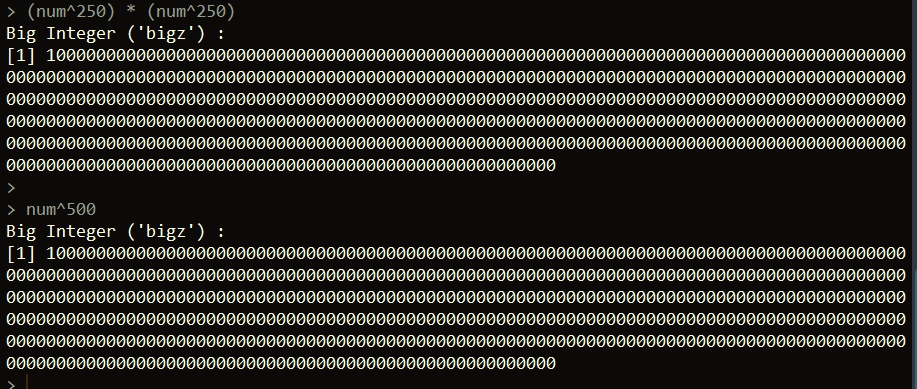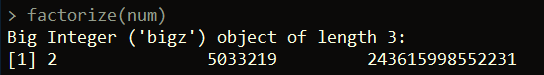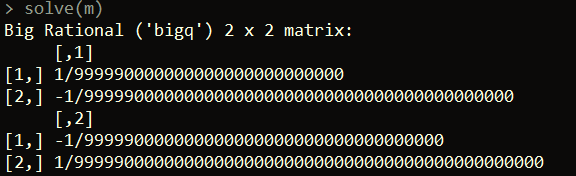# Really large numbers in R

August 15, 2019
By

Want to share your content on R-bloggers? click here if you have a blog, or here if you don't.This post will discuss ways of handling huge numbers in R using the gmp package.

## The gmp package

The gmp package provides us a way of dealing with really large numbers in R. For example, let’s suppose we want to multiple 10250 by itself. Mathematically we know the result should be 10500. But if we try this calculation in base R we get Inf for infinity.

```num = 10^250

num^2 # Inf

```

However, we can get around this using the gmp package. Here, we can convert the integer 10 to an object of the bigz class. This is an implementation that allows us to handle very large numbers. Once we convert an integer to a bigz object, we can use it to perform calculations with regular numbers in R (there’s a small caveat coming).

```library(gmp)

num = as.bigz(10)

(num^250) * (num^250)

# or directly 10^500
num^500

```One note that we need to be careful about is what numbers we use to convert to bigz objects. In the example above, we convert the integer 10 to bigz. This works fine for our calculations because 10 is not a very large number in itself. However, let’s suppose we had converted 10250 to a bigz object instead. If we do this, the number 10250 becomes a double data type, which causes a loss in precision for such a number. Thus the result we see below isn’t really 10250:

```num = 10^250

as.bigz(num)

num

```A way around this is to input the number we want as a character into as.bigz. For example, we know that 10250 is the number 1 followed by 250 zeros. We can create a character that represents this number like below:

```num = paste0("1", paste(rep("0", 250), collapse = ""))

```

Thus, we can use this idea to create bigz objects:

```as.bigz(num)

```In case you run into issues with the above line returning an NA value, you might want to try turning scientific notation off. You can do that using the base options command.

```options(scipen = 999)

```

If scientific notation is not turned off, you may have cases where the character version of the number looks like below, which results in an NA being returned by as.bigz.

“1e250”

In general, numbers can be input to gmp functions as characters to avoid this or other precision issues.

## Finding the next prime

The gmp package can find the first prime larger than an input number using the nextprime function.

```num = "100000000000000000000000000000000000000000000000000"

nextprime(num)

```## Find the GCD of two huge numbers

We can find the GCD of two large numbers using the gcd function:

```num = "2452345345234123123178"
num2 = "23459023850983290589042"

gcd(num, num2) # returns 2

```

## Factoring numbers into primes

gmp also provides a way to factor numbers into primes. We can do this using the factorize function.

```num = "2452345345234123123178"

factorize(num)

```## Matrices of large numbers

gmp also supports creating matrices with bigz objects.

```num1 <- "1000000000000000000000000000"
num2 <- "10000000000000000000000000000000"
num3 <- "100000000000000000000000000000000000000"
num4 <- "100000000000000000000000000000000000000000000000"

nums <- c(as.bigz(num1), as.bigz(num2), as.bigz(num3), as.bigz(num4))

matrix(nums, nrow = 2)

```We can also perform typical operations with our matrix, like find its inverse, using base R functions:

```solve(m)

```## Sampling random (large) numbers uniformly

We can sample large numbers from a discrete uniform distribution using the urand.bigz function.

```urand.bigz(nb = 100, size = 5000, seed = 0)

```

The nb parameter represents how many integers we want to sample. Thus, in this example, we’ll get 100 integers returned. size = 5000 tells the function to sample the integers from the inclusive range of 0 to 25000 – 1. In general you can sample from the range 0 to 2size – 1.

The post Really large numbers in R appeared first on Open Source Automation.

R-bloggers.com offers daily e-mail updates about R news and tutorials about learning R and many other topics. Click here if you're looking to post or find an R/data-science job.
Want to share your content on R-bloggers? click here if you have a blog, or here if you don't.